# [Solved] Two Decimal Places

I want to make this texts shows the value with two decimal places.

Here’s their initial value.

I want it to show two decimal places (for ex. Instead of Attack Damage value is 1, 1.00 Instead.)
Hope someone helps me because this is crucial to my game for I will modify those variables and add 0.10 or 0.25 when the player gets power-ups so I badly need to make all variables shows with two decimal places. Thank you in advance!

When you modify the variables by 0.10 and 0.25, it will display the decimal places.

As far as forcing it to display 1.00 or 2.00, I’m not aware of any formula/expression that will force it to display decimals when it’s a full integer.

You might be able to do some events that check if there’s any remainder (mod(whatevernumbersetc,1)) and if there’s no remainder, append the text with “.00” or something?

2 Likes

Hi, firstly try putting a decimal point (like 1.0) on the variable.
Then, you can use SubStr().
For example if you want to show Attack_Damage with 2 decimal places on a text, use:
`SubStr(ToString(Attack_Damage),0,4)`
It should appear as 1.00 . Note that the actual variable is still 1.000000000, it just changes how you display it on the text.

By the way, check out these two cute buttons, they will tell you every function available. They are great!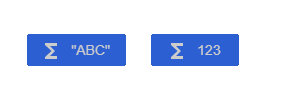Ref: Expressions reference [GDevelop wiki]

4 Likes

I try to use the SubStr just like you did.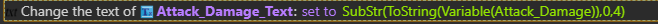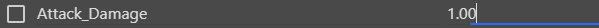Unfortunately, it’s not working. It shows only “1”.

You can try Silver_Streak’s suggestion, it works.

1. Make another event
2. For condition, use “Compare two numbers”
3. For action, use ToString(Variable(Attack_Power)) + “.00” on your text

How it works: Modulus checks for remainder of division. 1 / 1 = 1 so 0 remainder = 0 in modulus result.
Then, we use ToString to display the variable and + “.00” to display fake decimals at the end of the number.

The event looks like this: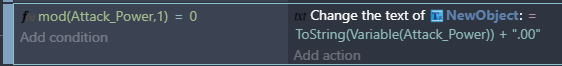It will display 1.00.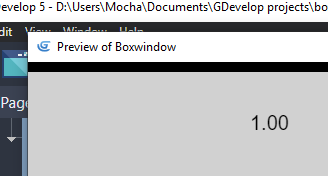2 Likes
3 Likes

Here’s a simple solution that uses a little snip of JavaScript. You could put this into a function, and call it up as needed. :

Which results in :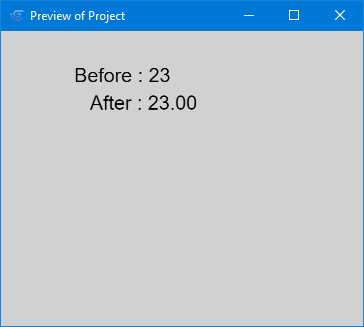The code :

`var original = runtimeScene.getVariables().get('Original').getAsNumber(); runtimeScene.getVariables().get('Modified').setString(original.toFixed(2));`

1 Like